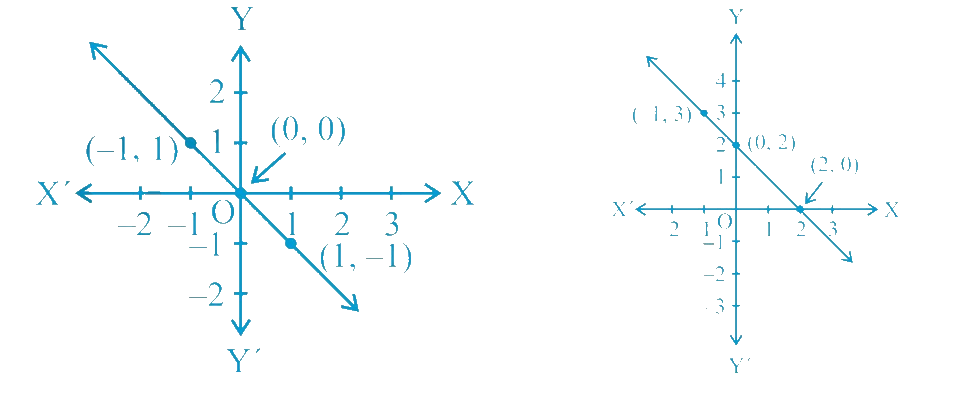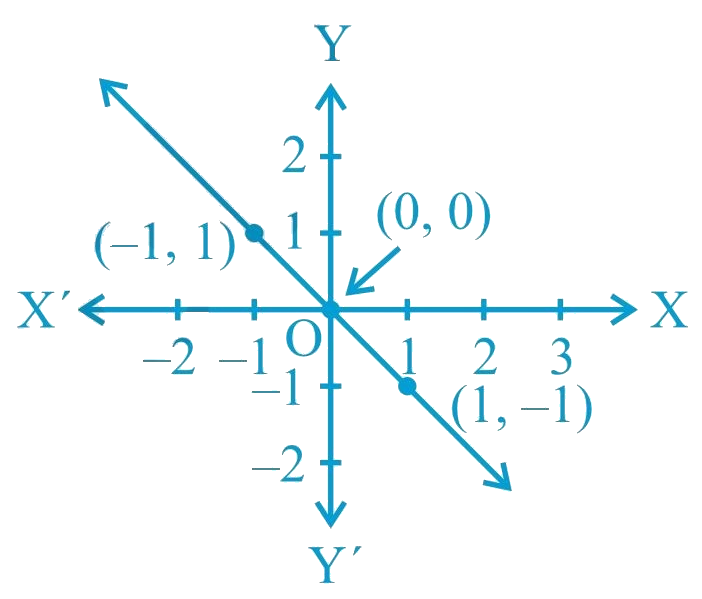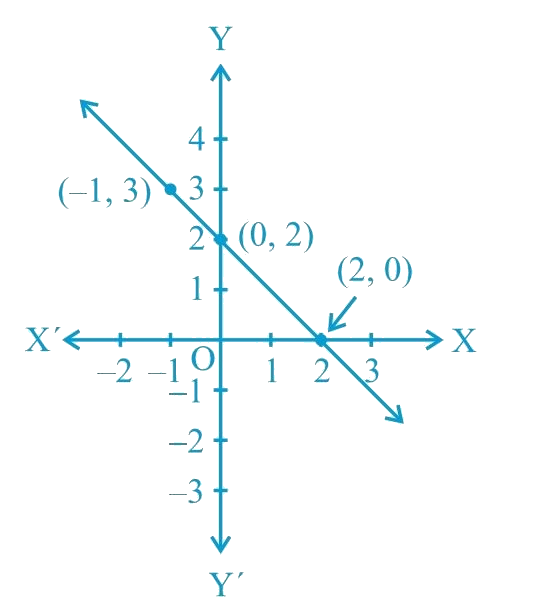# Ex.4.3 Q5 Linear Equations in Two Variables Solution - NCERT Maths Class 9

Go back to  'Ex.4.3'

## Question

From the choices given below, choose the equation whose graphs are given in the following figures.

 For the first figure For the second figure (i) $$y = x$$ (i) $$y = x + 2$$ (ii) $$x + y = 0$$ (ii) $$y = x − 2$$ (iii) $$y = 2x$$ (iii) $$y = − x + 2$$ (iv) $$2 + 3y = 7x$$ (iv) $$x + 2y = 6$$Video Solution
Linear Equations In Two Variables
Ex 4.3 | Question 5

## Text Solution

What is known?

Graph and equations

What is Unknown?

Given equations is of which graph .

Reasoning:

We can check by substituting coordinate values in given equations

Steps:

For the First Figure:• Points on the given line are $$(−1, 1)$$, $$(0, 0),$$ and $$(1, −1)$$.
• It can be observed that the coordinates of the points of the graph satisfy the equation $$x + y = 0$$.

Therefore, $$x + y = 0$$ is the equation corresponding to the graph as shown in the first figure.

Hence, (ii) is the correct answer.

For the Second Figure:• Points on the given line are $$(−1, 3)$$, $$(0, 2),$$ and $$(2, 0).$$
• It can be observed that the coordinates of the points of the graph satisfy the equation $$y = −x + 2$$. Therefore, $$y = −x + 2$$ is the equation corresponding to the graph shown in the second figure.Hence, (iii) is the correct answer.
Video Solution
Linear Equations In Two Variables
Ex 4.3 | Question 5

Learn from the best math teachers and top your exams

• Live one on one classroom and doubt clearing
• Practice worksheets in and after class for conceptual clarity
• Personalized curriculum to keep up with school